NOTIFICATION: These examples are provided for educational purposes. The use of this code and/or information is under your own responsibility and risk. The information and/or code is given ‘as is’. I do not take responsibilities of how they are used.

Poly-alphabetic Cipher

In the previous posting, we say that the mono-alphabetic cipher instead of shifting the alphabet a number of letters (Caesar cipher), its substitute each letter arbitrarily by mapping the plaintext letter map to a random arranged ciphertext. The only requirement for the ciphertext was that the letters must not be repeated. Now we are going to see a cipher that uses a set of related mono-alphabetic rules plus a key to determine which rule will be use to perform a transformation.

Vigenère Cipher

Encryption:

This cipher is similar to the Caesar cipher for the use of the 26 letters alphabet with the only different that we create a table in which:

1. The columns represent the plain text
2. The rows represent the key
3. The alphabet inside the table is shifted to the right one letter one time for each letter of the alphabet key.

To be more clear, let take a quick look of the Caesar cipher table: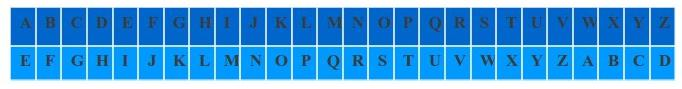In this example, We started the alphabet on the letter ‘E’ because the key was 5.

Now, the Vigenère Cipher will apply this shifting 26 times, one time per row, for each letter of the alphabet that correspond to the key as follow: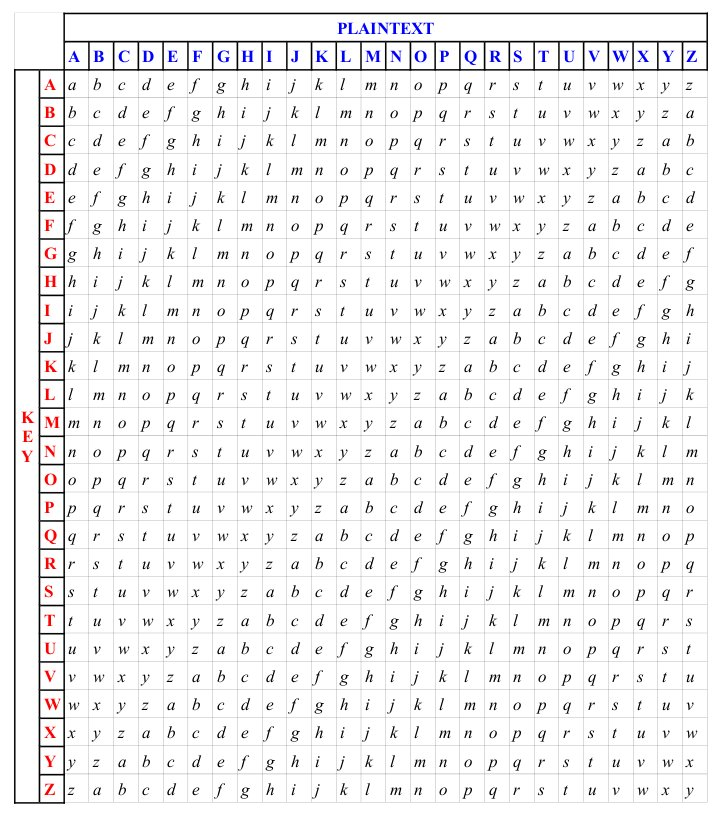Lets say that you have the following key “THIS  MESSAGE WAS FOR YOU”, and your key is “HELLO” then using the table: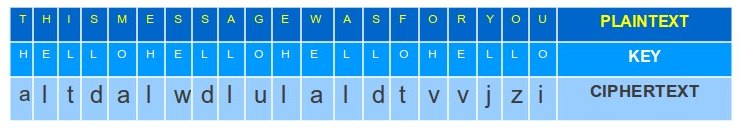From a mathematical point of view we have:

1. Lets assume that we take the letters of the alphabet from A to Z and be replace them with number starting from 0, for example: A = 0, B = 1, …, Z = 25.
2. Since we have 26 letters in the alphabet, lets perform module of 26 on this equation.
3. If ‘i’ is the letter position, P indicate the plaintext, K indicate the key, and C indicate the ciphertext then:Decryption

For decryption we only need to use a letter of the key to identify the row and the letter of the ciphertext in the row to identify the column, the letter designated to the column give us the plaintext letter.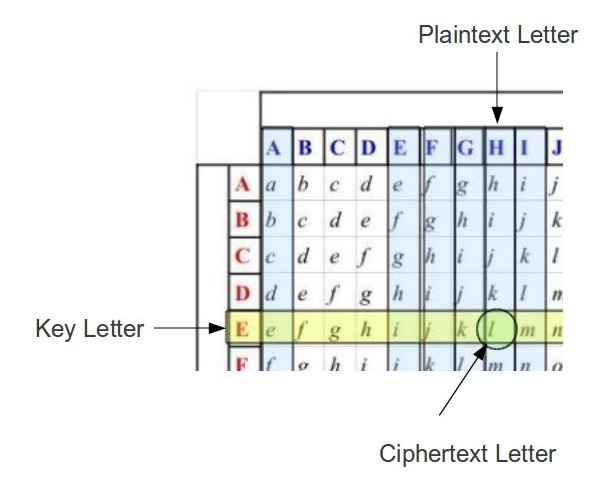From a mathematical point of view we have:

1. Lets assume that we take the letters of the alphabet from A to Z and be replace them with number starting from 0, for example: A = 0, B = 1, …, Z = 25.
2. Since we have 26 letters in the alphabet, lets perform module of 26 on this equation.
3. If ‘i’ is the letter position, P indicate the plaintext, K indicate the key, and C indicate the ciphertext then:Security

This cipher is not secure. If two or more sequences are identical inside the plaintext, we run the risk that identical ciphertext sequence will be generated. The attacker can use these repetition in the ciphertext to make a deduction about what is the plaintext. The more plaintext is needed to encrypt, the more chances that the ciphertext can be broken or the key found.

As an example, lets assume we have the following:
Plaintext:   WE RUN WHEN WE WERE DISCOVER BY THEM
Key:             RUNNING NO RUNNING NO RUNNING NO

This would give us a ciphertext in which we can spot the repetitions: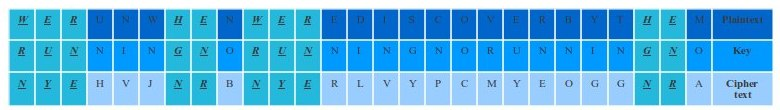The only way around this problem is by using the Autokey cipher.

Autokey Cipher

An auto-key cipher is the concept of generating a key that does not have a repetition cycle.

Instead of having a plaintext and a key such as this example:
Plaintext:   WE RUN WHEN WE WERE DISCOVER BY THEM
Key:             RUNNING NO RUNNING NO RUNNING NO

We could have the following key:
Plaintext:   WE RUN WHEN WE WERE DISCOVER BY THEM
Key:             RUNNING IS NOT THE SOLUTION THIS

This would give us a ciphertext with no repetitions: